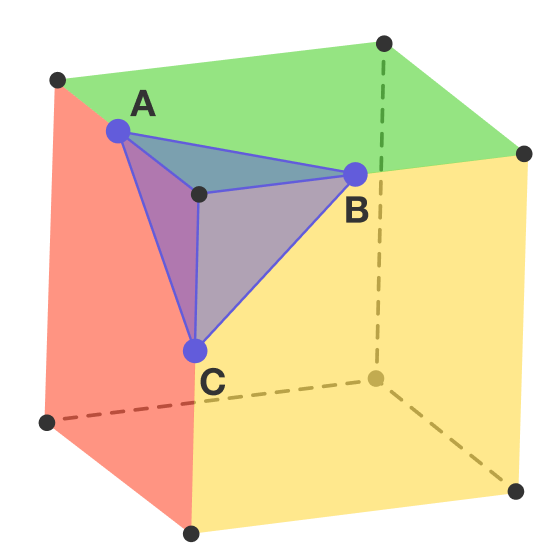# GRE Question

Geometry Level 1The volume of the cube shown above is 64. The vertices of triangle $ABC$ are the midpoints of the corresponding edges of the cube. What is the perimeter of triangle $ABC$ ?

×

Problem Loading...

Note Loading...

Set Loading...